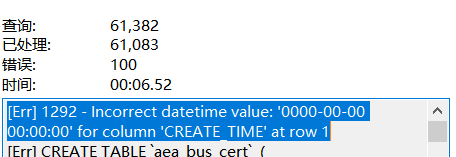4.22 错误优化策略

### 4.22.1 final无法帮助内联

``````public final String getUserName(){
}
``````

### 4.22.2 subString 内存泄露

``````private final char value[];
public String(char value[], int offset, int count) {
.....
this.value = Arrays.copyOfRange(value, offset, offset+count);
}
``````

### 4.22.3 循环优化

``````stratTime = System.nanoTime();
for (int i = 0; i < 100_000_00; i++) {
for (int j = 0; j < 10; j++) {

}
}
endTime = System.nanoTime();
System.out.println("外大内小耗时："+ (endTime - stratTime));
``````

``````stratTime = System.nanoTime();
for (int i = 0; i <10 ; i++) {
for (int j = 0; j < 10000000; j++) {
}
}
endTime = System.nanoTime();
System.out.println("外小内大耗时："+(endTime - stratTime));
``````

``````//ForDeadCodeTest.java
@Benchmark
public long test(Blackhole hole) {
long startTime = System.nanoTime();
int i=0,j=0;
for ( i = 0; i < 100_000_00; i++) {
for ( j = 0; j < 10; j++) {
hole.consume(j);
}
hole.consume(i);
}

Long endTime = System.nanoTime();
return endTime-startTime+i+j;
}

@Benchmark
public long tes2(Blackhole hole) {
long startTime = System.nanoTime();
int i=0,j=0;
for ( i = 0; i <10 ; i++) {
for ( j = 0; j < 100_000_00; j++) {
hole.consume(j);
}
hole.consume(i);
}
Long endTime = System.nanoTime();
return endTime-startTime+i+j;
}
//测试基准，空函数
@Benchmark
public long base(Blackhole hole) {
long startTime = System.nanoTime();

Long endTime = System.nanoTime();
return endTime-startTime;
}
``````

``````Benchmark                       Mode     Score    Units
``````

### 4.22.4 循环中捕捉异常

``````for(int i=0;i<size;i++){
try{

}catch(Exception ex){

}
}
``````

``````try{
for(int i=0;i<size;i++){

}
}catch(Exception ex){
}
``````blmius
2年前
MySQL:[Err] 1292 - Incorrect datetime value: ‘0000-00-00 00:00:00‘ for column ‘CREATE_TIME‘ at row 1Jacquelyn38
2年前
2020年前端实用代码段，为你的工作保驾护航Wesley13
1年前
java系统优化中的位操作优化Stella981
1年前
Opencv中Mat矩阵相乘——点乘、dot、mul运算详解Wesley13
1年前
P2P技术揭秘.P2P网络技术原理与典型系统开发Wesley13
1年前
00：Java简单了解Stella981
1年前
Android蓝牙连接汽车OBD设备
//设备连接public class BluetoothConnect implements Runnable {    private static final UUID CONNECT_UUID  UUID.fromString("0000110100001000800000805F9B34FB");Wesley13
1年前
Java性能优化Stella981
1年前Wesley13
1年前
MySQL部分从库上面因为大量的临时表tmp_table造成慢查询Wesley13
Lv1

1.2w

18

1.7k